Refer to our Texas Go Math Grade 1 Answer Key Pdf to score good marks in the exams. Test yourself by practicing the problems from Texas Go Math Grade 1 Lesson 12.3 Answer Key Add 3 Numbers.

Explore

Listen to the problem. Show two ways to group and add the numbers.Given,
By adding three with three we get six and then adding six with six we get twelve as the sum,
In the same way we can change the addends and can get the sum as the same,
By adding six with we get nine and then adding nine with three we get twelve as the sum.

FOR THE TEACHER • Read the following problem. There are 3 children at one table. There are 6 children at another table. There are 3 children in line. How many children are there?
Given,
There are three children at one table,
There are six children at another table,
There are three children in line,
So by adding three with six we get nine and then by adding nine with three we get twelve.
So there are total of twelve children.

Math Talk
Mathematical Processes

Describe the two ways you grouped the numbers to add.

Model and Draw

You can group the addends in any order, and in different ways to find the sum.Given,
By adding eight with two we get ten, and then by adding ten with six we get sixteen,
In the same way, we can change the addends and can get the same sum,
By adding six with two we get eight and then by adding eight with eight we get the sum as sixteen.

Share and Show

Choose a strategy. Circle two addends to add first. Write the sum. Then find the total sum. Then use a different strategy and add again.

THINK: Use count on. doubles, doubles plus one, doubles minus one, or make a ten to add.

Question 1.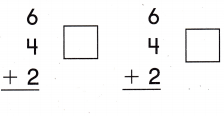Given,
By adding six with two we get eight, and then add eight with four we get twelve as the sum,
In the same way, we can change the addends and the get the same sum,
By adding four with two we get six and then add six with six we get twelve as the sum,

Question 2.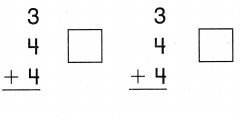Given,
By adding three with four we get seven, and then adding seven with four we get eleven as the sum,
In the same way, we can change the addends to get the sum as the same,
By adding four with four we get eight, and then adding eight with three we get eleven as the sum,

Question 3.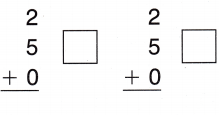Given,
By adding two with zero we get two, anything with zero we get the same number as the sum and then adding two with five we get seven as the sum,
In the same way, we can change the addends and get the same sum,
By adding five with zero we get five, and then adding five with two we get seven as the sum,

Question 4.Given,
By adding five with five we get ten, and then adding ten with four we get fourteen as the sum,
In the same way, we can change the addends and get the sum as the same.
By adding four with five we get nine, and by adding nine with five we get fourteen as the sum.

Problem Solving

Choose a strategy. Circle two addends to add first Write the sum.

Question 5.Given,
By adding eight with we get ten,
and then adding ten with two we get twelve,

Question 6.Given,
By adding six with zero we get six,
And then adding six with eight we get fourteen,

Question 7.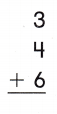Given,
By adding three with four we get seven,
And then adding seven with six we get thirteen,

Question 8.Given,
By adding two with three we get five,
And then adding five with seven we get twelve,

Question 9.Given,
By adding seven with seven we get fourteen,
And then adding fourteen with two we get sixteen,

Question 10.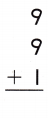Given,
By adding nine with nine we get eighteen,
And then adding eighteen with one we get nineteen,

Question 11.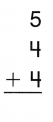Given,
By adding five with four we get nine,
And then adding nine with four we get thirteen,

Question 12.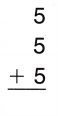Given,
By adding five with five we get ten,
And then adding ten with five we get fifteen,

Question 13.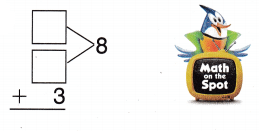Given,
The missing addends are four and four,
By adding four with four we get eight,
By adding eight with three we get eleven,
So the missing addends are four and four.

Question 14.Given,
The missing addends are four and three,
By adding four with three we get seven,
By adding seven with three we get ten,
So the missing addends are four and three.

Draw a picture. Complete the number sentence.

Question 15.
H.O.T. Multi-Step Kathy sees 13 fish in the tank. 6 fish are gold. The rest are blue or red. How many of each could she see?________ + _____ + 6 = 13 fish
Answer: There must be four blue fishes and three red fishes

Explanation:
Given,
Kathy sees thirteen fishes in the tank,
Six fishes are gold,
So the remaining fishes are blue or red fishes,
By subtracting six from thirteen we get seven,
So the missing addends are four and three,
By adding four with three we get seven.

Question 16.
Apply Jerry has 4 red caps. He has 6 green caps. He has 3 blue caps. How many caps does Jerry have?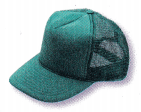(A) 14
(B) 10
(C) 13
Given,
Jerry has four red caps,
Six green caps,
And three blue caps,
So by adding four with six we get ten,
By adding ten with three we get thirteen,
So Jerry has a total of thirteen caps.

Question 17.
Ty sees 5 blue kites. He sees 7 red kites. He sees 4 purple kites. How many kites does he see?(A) 16
(B) 12
(C) 19
Given,
Ty sees five blue kites,
Seven red kites,
And four purple kites,
So by adding five with seven we get twelve,
By adding twelve with four we get sixteen,
So Ty sees a total of sixteen kites.

Question 18.
Multi-Step Ben has 7 white blocks. He has 7 blue blocks. He has some red blocks. Ben has 16 blocks in all. How many red blocks does Ben have?(A) 1
(B) 4
(C) 2
Given,
Ben has seven white blocks,
Seven blue blocks,
Some red blocks with total of sixteen blocks,
By adding seven with seven we get fourteen,
So there is total of sixteen blocks,
By subtracting fourteen from sixteen we get two,
Therefore, there are two red blocks remaining.

Question 19.
Texas Test Prep What is the sum of 7 + 4 + 3?
(A) 7
(B) 13
(C) 14
Given,
By adding seven with four we get eleven,
By adding eleven with three we get fourteen,

TAKE HOME ACTIVITY • Have your child explain how he or she can odd the numbers 3, 2, and 5.

### Texas Go Math Grade 1 Lesson 12.3 Homework and Practice Answer Key

Choose a strategy. Circle two addends to add first. Write the sum. Then find the total sum. Then use a different strategy and add again.

Question 1.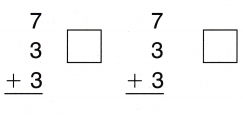Given,
By adding seven with three we get ten,
Then by adding ten with three we get thirteen,
Even after changing the addends we get the same sum,
By adding three with three we get six,
Then by adding six with seven we get thirteen,

Question 2.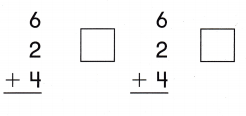Given,
By adding six with two we get eight,
Then by adding eight with four we get twelve,
Even after changing the addends we get the same sum,
By adding two with four we get six,
Then after by adding six with six we get twelve,

Question 3.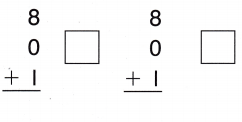Given,
By adding eight with zero we get eight,
Then by adding eight with one we get nine,
Even after changing the addends we get the same sum,
By adding one with zero we get one,
Then by adding one with eight we get nine as the sum,

Question 4.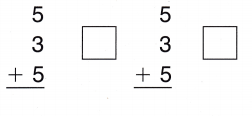Given,
By adding five with three we get eight,
Then by adding eight with five we get thirteen,
Even after changing the addends we get the same sum,
By adding five with we get ten,
Then by adding ten with three we get thirteen,

Problem Solving

Draw a picture. Complete the number sentence.

Question 5.
Todd has 5 green paper clips. He has 2 blue paper clips. He has 8 red paper clips. How many paper clips does Todd have?
__________ + _________ + = ________ paper clips
Given,
Todd has five green paper clips,
Two blue paper clips,
And eight red paper clips,
So by adding five with two we get seven,
Then after adding seven with eight we get fifteen,
So Todd has a total of fifteen paper clips.

Lesson Check

Question 6.
Zeb counts 2 black cars. He counts 7 grey cars. He counts 1 red car. How many cars did Zeb count?
(A) 8
(B) 10
(C) 9
Given,
Zeb counts two black cars,
Seven grey cars,
And one red car,
So by adding two with seven we get nine,
Then by adding nine with one we get ten,
So Zeb counts a total of ten cars.

Question 7.
Abby gave out paper party hats. She gave out 2 silver hats. She gave out 4 pink hats. Then she gave out 6 yellow hats. How many hats did Abby give out?(A) 12
(B) 6
(C) 10
Given,
Abby gave two silver hats,
Four pink hats,
And six yellow hats,
So by adding two with four we get six,
Then after adding six with six we get twelve,
So Abby gave out a total of twelve hats.

Question 8.
Multi-Step Lana has 16 balloons. She has 4 blue balloons. She has some green balloons. She has 5 purple balloons. How many green balloons does Lana have?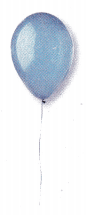(A) 11
(B) 9
(C) 7
Given,
Lana has sixteen balloons,
Four blue balloons,
Some green balloons,
And five purple balloons,
So by adding four with five we get nine,
By subtracting nine from sixteen we get seven,
Seven is the remaining count of green balloons,
Lana has seven green balloons.

Question 9.
What is the sum of 5 + 9 + 5?
(A) 13
(B) 19
(C) 10